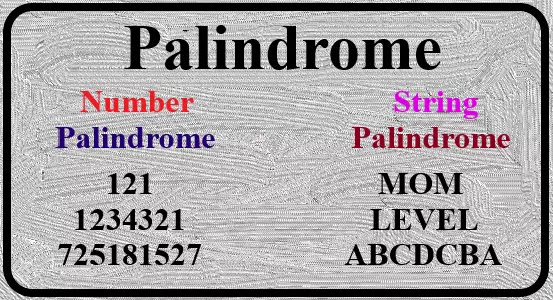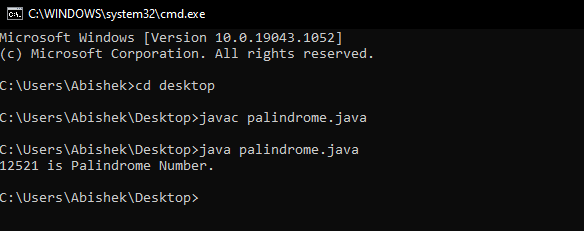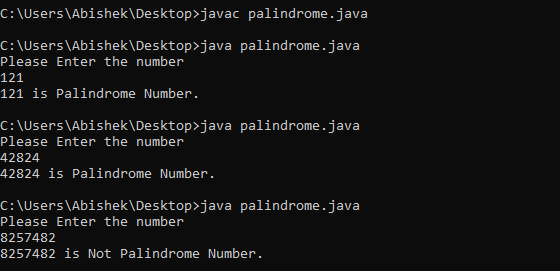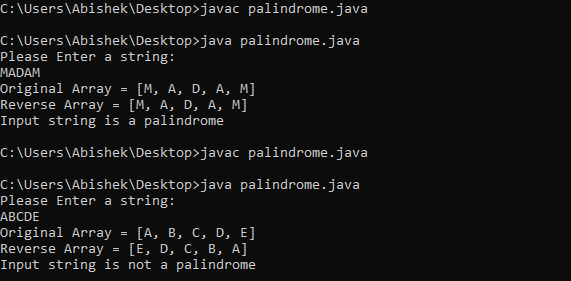# Palindrome Program in Java

### What is Palindrome number?

A number is said to be a palindrome if the number is same after reverse. For example - 11, 121, 999, 45654.

### What is Palindrome String?

A String is said to be a palindrome if the String is same after reverse. For example - MOM, MADAM, LEVEL, MALAYALAM.There are many ways to implement the Palindrome program in Java. But today we will discuss some of their important ways with example.

So let's Start..

## Check if a number is palindrome or not using for loop in java.

Algorithm:
• Take the number that needs to be checked for Palindrome.
• Store the number into temporary variable.
• Reverse the number (using for loop).
• Compare reverse number with temporary number.
• If both numbers are the same, print "Given Number is palindrome".
• Else, print "Given number is not palindrome".
• ``````
class Palindrome
{
public static void main(String args[])
{
int remainder;
int sum=0;
int number = 12521;//This is the number that needs to be checked
int temp = number; // holding input number in temp variable.
for( ; number!=0;number/=10 ) //number/=10, means number=number/10;
{
remainder = number%10;  //getting remainder.
sum = (sum*10)+remainder; // getting reverse.
}
if(temp == sum) //Comparing original number(temp) with reversed number(sum).
System.out.println(temp+" is Palindrome Number.");
else
System.out.println(temp+" is Not Palindrome Number.");
}
}
``````## Check whether input number is Palindrome or not using while loop in java.

Algorithm:
• Take the number that needs to be checked for Palindrome.
• Store the number into temporary variable.
• Reverse the number(using while loop).
• Compare reverse number with temporary number.
• If both numbers are the same, print "Input number is a palindrome".
• Else print "Input number is not a palindrome".
• ``````import java.util.*;
class Palindrome{
public static void main(String args[]){
int r,sum=0;
Scanner scan = new Scanner(System.in);
int number = scan.nextInt(); // taking input from user.
int temp = number; // holding input number in temp variable.
while(number>0){
r = number%10;  //getting remainder.
sum = (sum*10)+r; // getting reverse.
number = number/10;
}
if(temp == sum) //Comparing original number(temp) with reversed number(sum).
System.out.println(temp+" is Palindrome Number.");
else
System.out.println(temp+" is Not Palindrome Number.");
}
}
``````## Check given String is palindrome or not in java using array.

Example -1
Algorithm:
• Convert the given string into a character array.
• Hold this array in temporary variable.
• Reverse the array.
• Compare the original array and the reversed array.
• If both strings are the same, print "Given string is palindrome".
• Else, print "Given number is not palindrome".
• ``````
import java.util.Arrays;
import java.util.Scanner;
class Palindrome {
public static void main(String args[]) {

Scanner scan = new Scanner(System.in);
String str = scan.nextLine();
char[] chArray = str.toCharArray();//toCharArray() method is used to convert string to character array.
int size = chArray.length;
char [] originalArray = Arrays.copyOf(chArray,size);//Arrays.copyOf() method is used to copy the array.

for (int i = 0; i < size / 2; i++)
{
char temp = chArray[i];
chArray[i] = chArray[size-i-1];
chArray[size-i-1] = temp;
}
System.out.println("Original Array = "+Arrays.toString(originalArray));
System.out.println("Reverse Array = "+Arrays.toString(chArray));

if(Arrays.equals(chArray, originalArray))//Arrays.equals() method has used to check whether two arrays are equal.
{
System.out.println("Input string is a palindrome");
} else {
System.out.println("Input string is not a palindrome");
}
}
}

``````## Check given String is palindrome or not in java using array(Another way).

Example -2
Algorithm:
• Convert the given string into a character array.
• Hold this array in temporary variable.
• Reverse the array.
• Compare the original array and the reversed array.
• If both strings are the same, print "Given string is palindrome".
• Else, print "Given number is not palindrome".
• ``````
import java.util.Arrays;
import java.util.Scanner;
class Palindrome {
public static void main(String args[]) {

Scanner scan = new Scanner(System.in);
String str = scan.nextLine();
char[] chArray = str.toCharArray();//toCharArray() method is used to convert string to character array.
int size = chArray.length;
char [] originalArray = Arrays.copyOf(chArray,size);//Arrays.copyOf() method is used to copy the array.

for (int i = 0; i < size / 2; i++)
{
char temp = chArray[i];
chArray[i] = chArray[size-i-1];
chArray[size-i-1] = temp;
}
System.out.println("Original Array = "+Arrays.toString(originalArray));
System.out.println("Reverse Array = "+Arrays.toString(chArray));

if(Arrays.equals(chArray, originalArray))//Arrays.equals() method has used to check whether two arrays are equal.
{
System.out.println("Input string is a palindrome");
} else {
System.out.println("Input string is not a palindrome");
}
}
}

``````

## Check if a string is palindrome or not in java using reverse method.

Algorithm:
• Get the String to check for palindrome.
• Reverse the string (using string reverse function).
• Compare the Original string with reversed string.
• If both strings are the same, print "This is palindrome string".
• Else, print "This is not palindrome string".
• ``````import java.util.*;
class Palindrome{
public static void main(String args[]){
Scanner scan = new Scanner(System.in);
System.out.println("Enter a string to check if it is a palindrome:");
String input = scan.nextLine();
String reverse = new StringBuffer(input).reverse().toString();//The reverse() method reverses an array in place
if (input.equals(reverse))
{
System.out.println("Yes, it is a palindrome");
}else{
System.out.println("No, it is not a palindrome");
}
}
}

``````

This is all about palindrome, in this article we have discussed what is palindrome number, what is palindrome string, and we also have discussed the code that, how to check palindrome number in java, how to check whether a string is palindrome or not with meaningful example. We hope you understand well. If you have any doubts, please comment in the comment box.